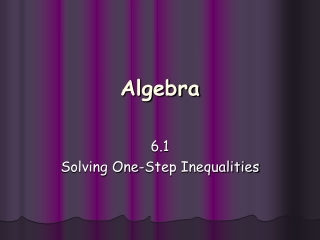DownloadDownload PresentationAlgebra

# Algebra

Télécharger la présentation## Algebra

- - - - - - - - - - - - - - - - - - - - - - - - - - - E N D - - - - - - - - - - - - - - - - - - - - - - - - - - -
##### Presentation Transcript

1. Algebra 6.1 Solving One-Step Inequalities

2. Two Mistakes on This Slideshow • See if you can spot them!

3. -5 -4 -3 -2 -1 0 1 2 3 4 5 6 7 x ≤ 4 The graph of an inequality is the set of points on a number line that are solutions. The graph is a boundary point and a line with an arrow pointing left or right.

4. -5 -4 -3 -2 -1 0 1 2 3 4 5 6 7 x > 2 A hollow point means that the number is not included in the set of solutions. Use a hollow point to graph < and > inequalities.

5. -5 -4 -3 -2 -1 0 1 2 3 4 5 6 7 x ≥ -3 A solid point means that the number is included in the set of solutions. Use a solid point to graph ≤ and ≥ inequalities.

6. -5 -4 -3 -2 -1 0 1 2 3 4 5 6 7 < x -1 The arrow points left for < and ≤ . The arrow points right for >and ≥ . The direction of the arrow tip is the same as the direction of the inequality symbol when the variable is on the left side.

7. Solve inequalities just as you would linear equations in one variable. Isolate the variable. Then graph the solution. x - 5 > -4 + 5 +5 3 x > 1 -1 0 1 2 4 15a ≤ -60 15 15 -7 -6 -5 -4 -3 -2 a ≤ -4

8. The only difference between solving an inequality and an equation is what happens when both sides are multiplied or divided by a negative value. 10 > 5 (2) (2) Multiply by positive 20 > 10 Inequality stays true 20 > 10 (-2) (-2) Multiply by negative -40 > -20 Inequality not true < Reverse direction of inequality symbol -40 -20

9. When solving an inequality, REVERSE THE DIRECTION OF THE INEQUALITY SYMBOL if you multiply or divide by a negative value. -¾a ≥ 6 Multiply by -4/3 Reverse direction of inequality symbol ≤ a -8

10. Solve the following inequalities and graph each solution. ¼x > -8 x > -32 -34 -33 -32 -31 -30 -29 a ≤ 50 47 48 49 50 51 52

11. Solve the following inequalities and graph each solution. Write the variable on the left in your solution. 42 > x x < 42 39 40 41 42 43 44 -3 < x x > -3 2 > -1 - x -5 -4 -3 -2 -1 0

12. Homework Pg. 337 #31 – 61 odd, 71-87